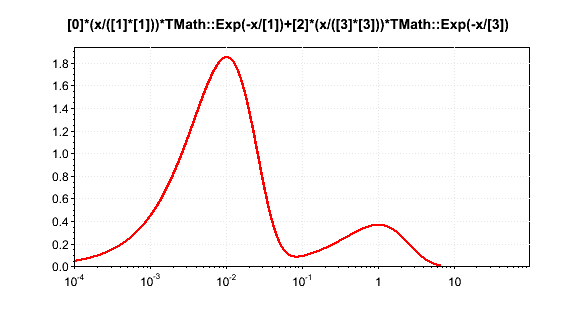# TF1 GetMaximum()

Hi there,

I’m trying to find the maximum of a TF1 having several maxima, the true one lying at small x (see plot below).
In that case, the GetMaximum() function does not return the true maximum unless I tell it where to seek (see code below),
which I don’t want to do since I have bunches of functions and I don’t feel like checking everything.
I guess this comes from the Brent’s method used (http://root.cern.ch/root/html520/src/TF1.cxx.html#NATlfB).
A temporary solution could be to get the max from all the GetMaximum(x,x+dx) ranging from xmin to xmax using a small log-equidistant binning.
But there might be a proper “Root way”…
Anyway, one should be aware using the GetMaximum() function could be risky.

Thanks for any tips,
Z

```Double_t xmin=1e-4, xmax=1e2, xmed=TMath::Sqrt(xmin*xmax), x1=0.01, x2=1. ; TF1 *fct = new TF1 ("fct"," * ( x / (*) ) * TMath::Exp(-x/) +  * ( x / (*) ) * TMath::Exp(-x/)",xmin,xmax) ; // Sum of 2 Maxwellian centered in x1 & x2 fct->SetParameter(0,0.05) ; fct->SetParameter(1,x1) ; fct->SetParameter(2,1. ) ; fct->SetParameter(3,x2) ; fct->SetNpx(100000) ; fct->Draw() ; printf("\nmax(%e,%e) = %e\n",xmin,xmax,fct->GetMaximum()) ; printf("\nmax(%e,%e) = %e\n",xmin,xmed,fct->GetMaximum(xmin,xmed)) ; ```Thanks for reporting this problem. A new algorithm was introduced after version 5.18 (where the answer was correct). We will investigate and improve this special case.

Rene

A similar issue with a Bethe-Bloch like function…

``` TF1 * f_BB = new TF1 ("f_BB","( 1. / ((x*x)/(1.+(x*x))) ) * ( TMath::Log((x*x)/1e-4) - ((x*x)/(1.+(x*x))) )", 1e-3,1e+5) ; f_BB->Draw() ; gPad->SetLogy() ; gPad->SetLogx() ; printf ( "\nmin [xmin;xmax] : x=%e ; y=%+e " ,f_BB->GetMinimumX() ,f_BB->GetMinimum() ) ; printf ( "\nmax [xmin;xmax] : x=%e ; y=%+e >> ISSUE" ,f_BB->GetMaximumX() ,f_BB->GetMaximum() ) ; printf ("\n\nmin [1e-1;xmax] : x=%e ; y=%+e >> ISSUE" ,f_BB->GetMinimumX(1e-1),f_BB->GetMinimum(1e-1)) ; printf ( "\nmax [1e-2;xmax] : x=%e ; y=%+e >> ISSUE\n\n",f_BB->GetMaximumX(1e-2),f_BB->GetMaximum(1e-2)) ; ```Cheers,
Z

Hi,

there is no magic in the algorithm to find the minimum/maximum. It scans first the function using n points to bracket the minimum/maximum. The n points is defined by the TF1::SetNpx (default is 100).
Unfortunately the maximum points allowed by TF1 is 100 000, so in your case it will not work.
Your first example works because 100 000 points are enough for that case.

A possible solution is to use directly the ROOT::Math::BrentMinimizer1D and set a larger number of points
(see attached macro)

One thing we could improve is to scan automatically in a log x grid, when the option logX is set, as it is done when plotting the function.
Also the maximum number of points could be relaxed to a larger value (like 10^6 or 7)

Best Regards

Lorenzo
findMaximum.C (801 Bytes)

Thanks Lorenzo.

[quote]One thing we could improve is to scan automatically in a log x grid, when the option logX is set, as it is done when plotting the function.[/quote]Or add a new argument (e.g. Bool_t logxsearch=kFALSE) in the corresponding TF1 methods if the function is not intended to be plotted.

[quote]Also the maximum number of points could be relaxed to a larger value (like 10^6 or 7)[/quote] Similarly, a method allowing the user to set this number would be nice.

Cheers,
Z

Thanks LorenzoCheers,
Z

BTW, to get a correct answer one has to explicitly draw the function, furthermore with gPad->SetLogx().

```TF1 * f_BB = new TF1 ("f_BB","( 1. / ((x*x)/(1.+(x*x))) ) * ( TMath::Log((x*x)/1e-4) - ((x*x)/(1.+(x*x))) )", 1e-3,1e+5) ; f_BB->Draw() ; gPad->SetLogx() ; printf ("\nxmin=%g",f_BB->GetMinimumX (f_BB->GetMaximumX(),f_BB->GetXmax(),1e-10,100,kTRUE)) ; printf ("\nymin=%g",f_BB->GetMinimum (f_BB->GetMaximumX(),f_BB->GetXmax(),1e-10,100,kTRUE)) ;```
Is there a way to avoid this?
Cheers,
Z